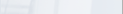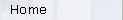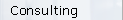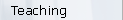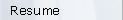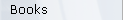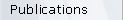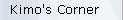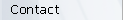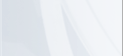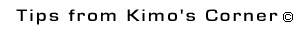Vapor Pressure, An Elegantly Simple Concept ©

In a later column the workings of a variety of cryopumps will be discussed. But first, we must understand the mechanisms by which they pump gases; that is, mechanisms defined as cryocondensation and cryosorption. These are elegantly simple mechanisms with complex-sounding names. Cryocondensation ties in closely with the vapor pressure of a gas, whereas cryosorption relates to the adsorption isotherm of the gas. We'll return in another article to a discussion of the latter pumping mechanism. This article deals with the concept of vapor pressure and how it relates to cryocondensation.

Cryocondensation involves the surface build-up of thick layers of either liquids or solids of a gas. For example, water vapor condensation on the outside of a chilled bottle of Corona beer is just a form of cryocondensation. Winter ice collecting on an automobile windshield is another form of water vapor cryocondensation. As long as the surface is sufficiently cold, and gas continues to impinge on the surface, the effective pumping of gas on the surface is independent of the amount of gas previously pumped thereon. Note, however, this says nothing about the rate that gas might be leaving the surface. We can define the rate, v  , at which gas molecules impinge on a surface by the following: 1

v  =
K1 x P molecules/sec-cm2                      (1)
K1 =
3.52 x 10 22 / (MT) ½
P =
the pressure in Torr
M =
the molucular weight of the gas
T =
the temperature of the gas in degrees Kelvin
R =

It seems reasonable to us all that the rate at which gas impinges on a surface is directly proportional to the pressure of that gas above the surface. However, what is less intuitive is that "gas", in the form of atoms or molecules, is also continuously leaving all surfaces. And, if there is a lot of a specific material on a surface, the rate at which that material leaves the surface is dependent on the vapor pressure of the material. For example, assume that you have a plate of clean copper on your workbench. The rate at which copper atoms are leaving or evaporating from the surface of the plate depends on the vapor pressure of copper at the given temperature. Now this doesn't necessarily mean that copper atoms are returning and impinging on the plate. Perhaps the vaporizing copper atoms are sticking on some other cool surface. By now, you are saying "so what Kimo?" Stick with me for a moment.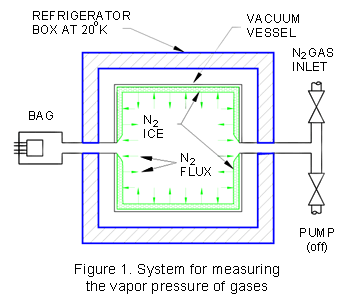Assume we have an experimental setup as illustrated in Fig. 1. Assume that the vacuum vessel contained within the refrigerator box is first evacuated and then cooled to 20 K. We then introduce nitrogen (N2) gas into the vacuum vessel to the point where a thick layer of N2"ice" builds up on the walls of the vacuum vessel, and then shut off the nitrogen valve. Shortly after shutting off the gas valve, the pressure in the box equilibrates. That is, the pressure neither increases nor decreases in the box, but stabilizes at a constant value. If the pressure is neither increasing nor decreasing, and (1) is valid, this means that for every molecule of N2 which leaves the ice surface, one must be impinging on and sticking to the ice. This one-for-one exchange process suggests that the void within the vessel must be saturated with N2 gas. It is! And, this is why under such circumstances we sometimes call that pressure in the vessel the saturation vapor pressure, or we can simply call it the vapor pressure of N2 at 20 K. The rate at which the N2 gas is impinging (and departing) the ice can be determined by use of (1). If we were to slightly increase the temperature of the vessel, the saturation vapor pressure would equilibrate at a higher value. If we were to reduce the temperature of the box, the vapor pressure of N2 gas would correspondingly decrease. Note that if we attempt to deduce the pressure within the box by using the room temperature Bayard-Alpert gauge (BAG) outside the box, we must take into account thermal transpiration effects. But, that's another subject.

Now, let's briefly squirt some more N2 gas into the vessel. The pressure will momentarily increase in the vessel so that the rate at which the gas molecules impinge on the surface will be greater than the rate at which they are departing. Eventually, however, the impingement and departure rates of the gas will again equilibrate, and the net flux of gas at the surface will again be zero. We say that the introduced gas has been cryocondensed, or the gas has been pumped by cryocondensation.

Now, let's continually introduce N2 gas into the vessel so that the pressure in the vessel is always greater than the vapor pressure of the ice. The gas will continually be pumped on the surface of the ice. If we know the N2 pressure in the vessel as a result of continually introducing gas, the rate of impingement of gas on the 20 K ice can be calculated with (1). On the other hand, the rate at which the gas is leaving the ice can also be predicted by (1) by plugging in the value of the vapor pressure of N2 at 20 K.

Note that if a cold piece of sheet metal was maintained at 20 K within a room temperature vacuum vessel, the sheet metal part would just as effectively cryocondense the gas as an equivalent surface area of the cold wall of our vessel in Fig. 1.

So, the main thrust of this discussion is that as long as the pressure of the N2 in the vessel is greater than the vapor pressure of N2, gas is being pumped on the ice surface. When the pressure of the N2 gas in the vessel approaches the vapor pressure of N2, the speed of the ice for N2 approaches zero as the subsequent net accumulation of gas on the surface will be zero. This is how cryocondensation works. It is important to note that the vapor pressures of all the gasses, except He, H2 and Ne, are £ 10 -11 Torr at 20K. That is, all those gases are very effectively cryocondensed at pressures ³ 10 -11 Torr.

It is obvious that knowing the vapor pressure of various gases is important when trying to predict how well a gas will be pumped (i.e., cryocondensed) on a surface of known temperature. Manipulation of an expression called the Clausius-Clapeyron equation leads to a simple algebraic equation expressing the vapor pressure, Pv, of all gases as a function of temperature, T, in degrees kelvin: 2

Log 10 Pv  =  A  -  B  x  T -1   +  C  x  Log 10  T       (2)

Constants A, B and C vary for the specific gas or material. Finding the values of these constants was a totally empirical process. That is, technicians, puttering around in laboratories throughout the world, made measurements of how different gases behaved as a function of temperature, compared results, and finally settled on appropriate values of A, B and C for different gases. Honig and Hook of RCA were the first to compile a comprehensive listing of these data for use by the scientific community. 3 If you know the values of A, B and C, and have the use of some spreadsheet program (e.g., Lotus, Excel, etc.) you can plot your own vapor pressure curves, or accurately calculate the vapor pressure of some gas at a specific temperature. Also, on request many gas suppliers will provide the values of A, B and C for some of the esoteric gases which they supply. The values of A, B and C for some of the common gases are given in Table 1. Have fun making your own vapor pressure curves.

Table 1. Clausius-Clapeyron constants
for some of the common gases.

 GAS A B C4 H2 3.881 42.730 0.50 D2 4.234 62.970 0.50 CH4 7.123 482.871 -- H2O 10.285 2637.006 -- Ne 6.889 109.270 -- N2 8.362 387.428 -- CO 8.732 445.831 -- O2 9.082 480.871 -- Ar 7.703 419.566 -- CO2 9.734 1349.809 -- Kr 7.797 581.967 -- Xe 7.775 801.641 --

The important issue here is that vapor pressure is a very simple concept. Gases behave according to Equation 1, and the constants A, B and C, therein, were found by folks around the world puttering in the lab and trying to fit their experimental results to (1).

Reference

1  Kimo M. Welch, Capture Pumping Technology, An Introduction, (Pergamon Press, Oxford 1991), p. 186.

2  Kimo M. Welch, Capture Pumping Technology, An Introduction, (Pergamon Press, Oxford 1991), p. 255.

3  R.E. Honig and H.O. Hook, "Vapor Pressure Data for Some Common Gases", RCA Review, 360 (1960).

4  T.J. Lee, "The Condensation of H2 and D2: Astrophysics and Vacuum Technology", J. Vac. Sci. Technol. 9(1), 257 (1972).